# Chapter 7 Chemical Quantities The Mole Collections of

• Slides: 47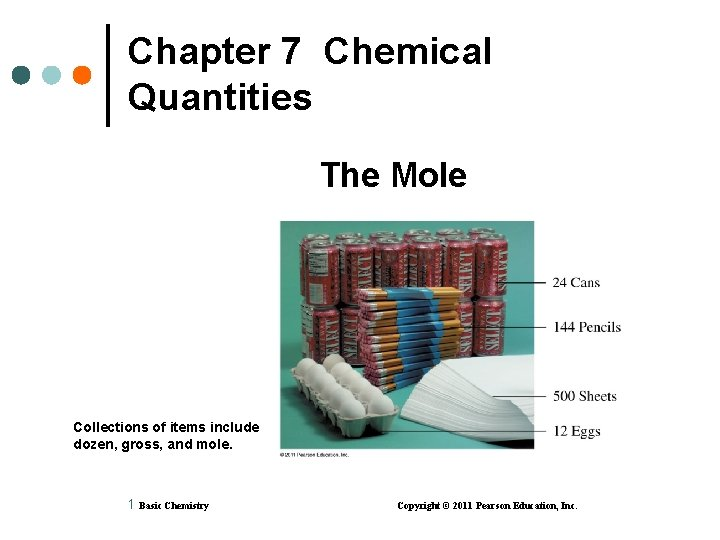Chapter 7 Chemical Quantities The Mole Collections of items include dozen, gross, and mole. 1 Basic Chemistry Copyright © 2011 Pearson Education, Inc.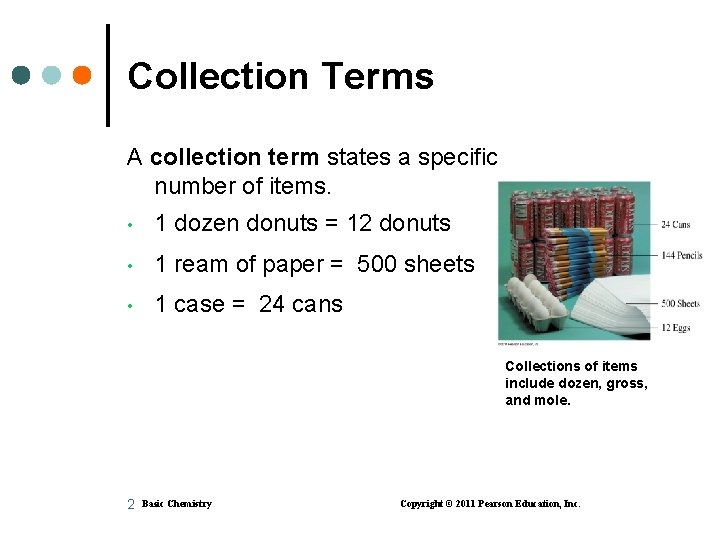Collection Terms A collection term states a specific number of items. • 1 dozen donuts = 12 donuts • 1 ream of paper = 500 sheets • 1 case = 24 cans Collections of items include dozen, gross, and mole. 2 Basic Chemistry Copyright © 2011 Pearson Education, Inc.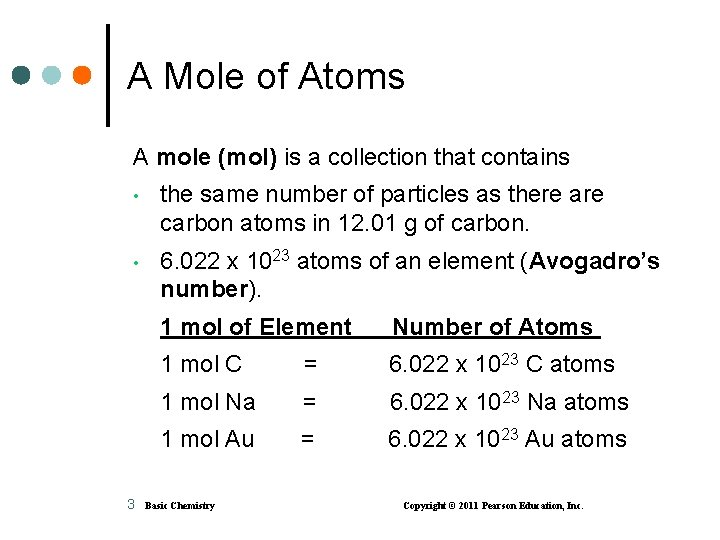A Mole of Atoms A mole (mol) is a collection that contains • the same number of particles as there are carbon atoms in 12. 01 g of carbon. • 6. 022 x 1023 atoms of an element (Avogadro’s number). 3 1 mol of Element Number of Atoms 1 mol C = 6. 022 x 1023 C atoms 1 mol Na = 6. 022 x 1023 Na atoms 1 mol Au = 6. 022 x 1023 Au atoms Basic Chemistry Copyright © 2011 Pearson Education, Inc.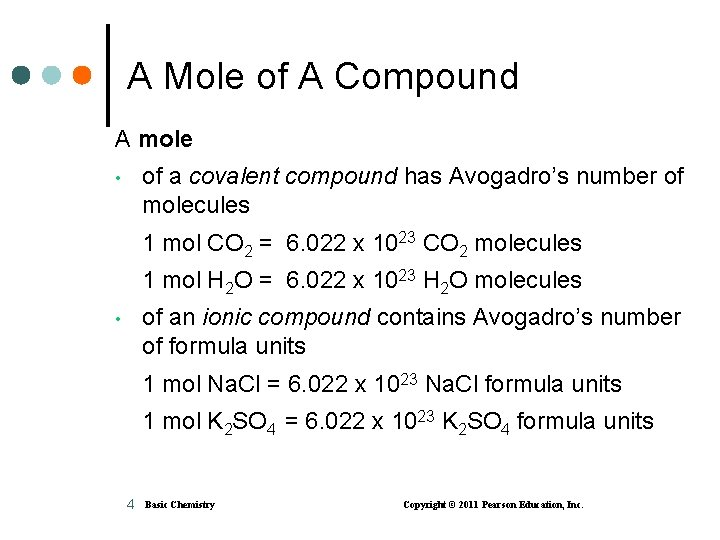A Mole of A Compound A mole of a covalent compound has Avogadro’s number of molecules • 1 mol CO 2 = 6. 022 x 1023 CO 2 molecules 1 mol H 2 O = 6. 022 x 1023 H 2 O molecules of an ionic compound contains Avogadro’s number of formula units • 1 mol Na. Cl = 6. 022 x 1023 Na. Cl formula units 1 mol K 2 SO 4 = 6. 022 x 1023 K 2 SO 4 formula units 4 Basic Chemistry Copyright © 2011 Pearson Education, Inc.Samples of One Mole Quantities 5 Basic Chemistry Copyright © 2011 Pearson Education, Inc.Avogadro’s Number Avogadro’s number (6. 022 x 1023) can be written as an equality and two conversion factors. Equality: 1 mol = 6. 022 x 1023 particles Conversion Factors: 6. 022 x 1023 particles and 1 mol 6. 022 x 1023 particles 6 Basic Chemistry Copyright © 2011 Pearson Education, Inc.Using Avogadro’s Number Avogadro’s number converts moles of a substance to the number of particles. How many Cu atoms are in 0. 50 mol of Cu? 0. 50 mol Cu x 6. 022 x 1023 Cu atoms 1 mol Cu = 3. 0 x 1023 Cu atoms 7 Basic Chemistry Copyright © 2011 Pearson Education, Inc.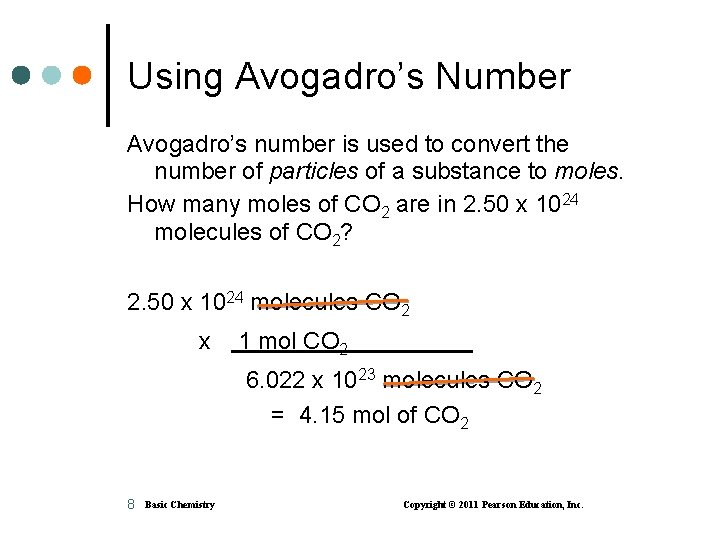Using Avogadro’s Number Avogadro’s number is used to convert the number of particles of a substance to moles. How many moles of CO 2 are in 2. 50 x 1024 molecules of CO 2? 2. 50 x 1024 molecules CO 2 x 1 mol CO 2 6. 022 x 1023 molecules CO 2 = 4. 15 mol of CO 2 8 Basic Chemistry Copyright © 2011 Pearson Education, Inc.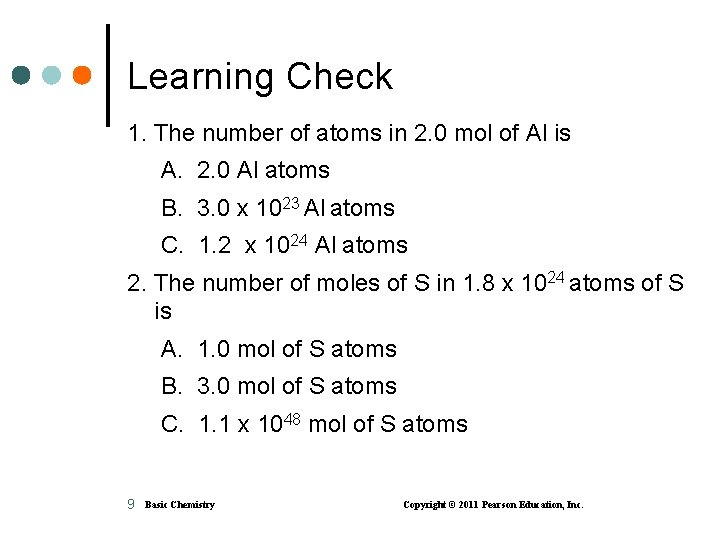Learning Check 1. The number of atoms in 2. 0 mol of Al is A. 2. 0 Al atoms B. 3. 0 x 1023 Al atoms C. 1. 2 x 1024 Al atoms 2. The number of moles of S in 1. 8 x 1024 atoms of S is A. 1. 0 mol of S atoms B. 3. 0 mol of S atoms C. 1. 1 x 1048 mol of S atoms 9 Basic Chemistry Copyright © 2011 Pearson Education, Inc.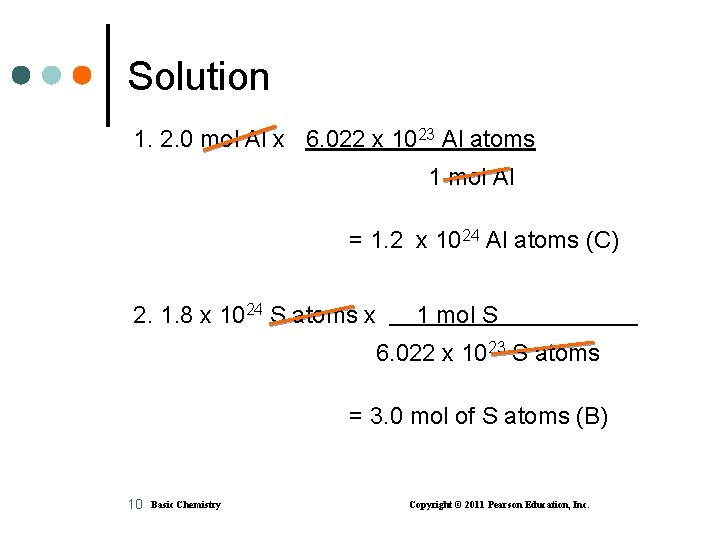Solution 1. 2. 0 mol Al x 6. 022 x 1023 Al atoms 1 mol Al = 1. 2 x 1024 Al atoms (C) 2. 1. 8 x 1024 S atoms x 1 mol S 6. 022 x 1023 S atoms = 3. 0 mol of S atoms (B) 10 Basic Chemistry Copyright © 2011 Pearson Education, Inc.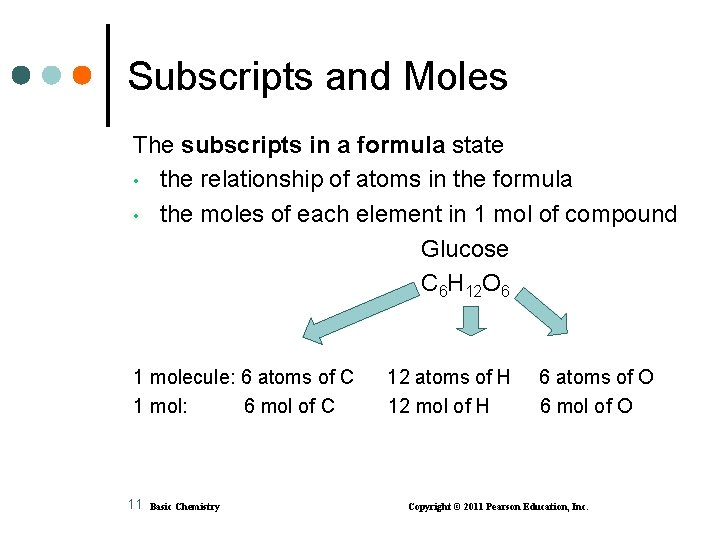Subscripts and Moles The subscripts in a formula state • the relationship of atoms in the formula • the moles of each element in 1 mol of compound Glucose C 6 H 12 O 6 1 molecule: 6 atoms of C 1 mol: 6 mol of C 11 Basic Chemistry 12 atoms of H 12 mol of H 6 atoms of O 6 mol of O Copyright © 2011 Pearson Education, Inc.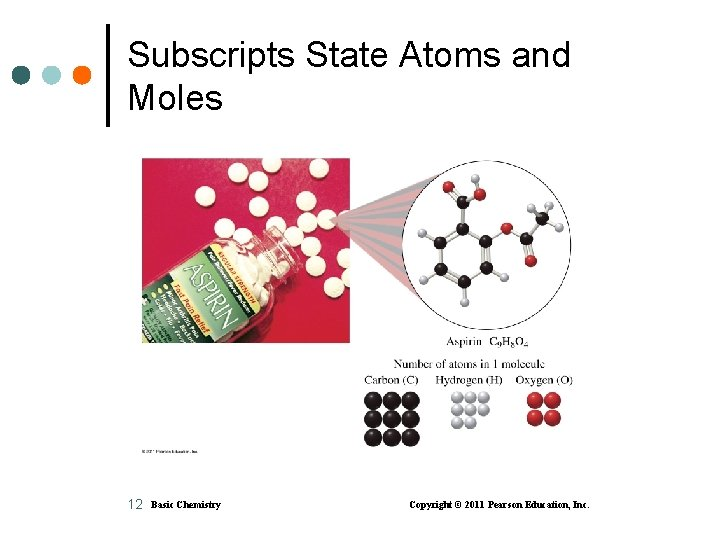Subscripts State Atoms and Moles 12 Basic Chemistry Copyright © 2011 Pearson Education, Inc.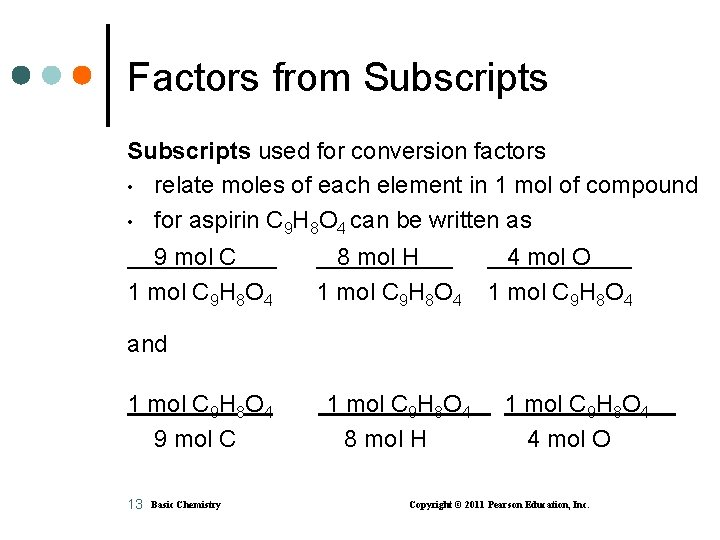Factors from Subscripts used for conversion factors • relate moles of each element in 1 mol of compound • for aspirin C 9 H 8 O 4 can be written as 9 mol C 1 mol C 9 H 8 O 4 8 mol H 1 mol C 9 H 8 O 4 4 mol O 1 mol C 9 H 8 O 4 and 1 mol C 9 H 8 O 4 9 mol C 13 Basic Chemistry 1 mol C 9 H 8 O 4 8 mol H 1 mol C 9 H 8 O 4 4 mol O Copyright © 2011 Pearson Education, Inc.Calculating Atoms or Molecules 14 Basic Chemistry Copyright © 2011 Pearson Education, Inc.Learning Check How many O atoms are in 0. 150 mol of aspirin, C 9 H 8 O 4? 15 Basic Chemistry Copyright © 2011 Pearson Education, Inc.Solution How many O atoms are in 0. 150 mol of aspirin, C 9 H 8 O 4? STEP 1 Given 0. 150 mol of C 9 H 8 O 4 Need molecules of C 9 H 8 O 4 STEP 2 Plan moles of aspirin 16 Basic Chemistry moles of O atoms of O Copyright © 2011 Pearson Education, Inc.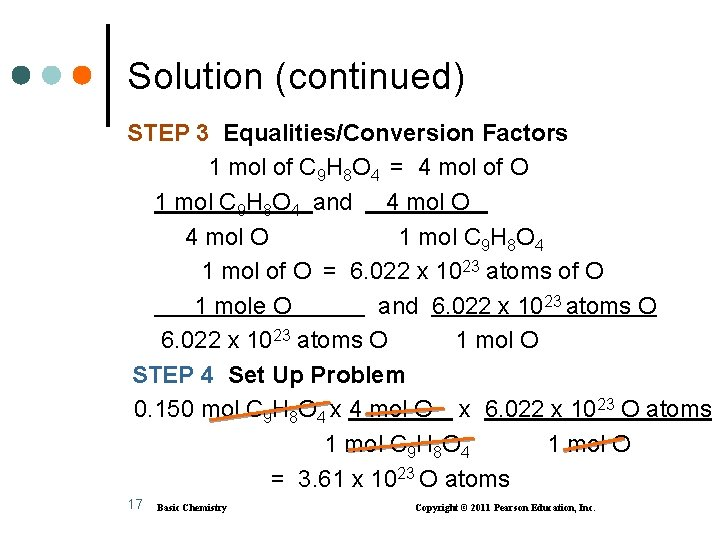Solution (continued) STEP 3 Equalities/Conversion Factors 1 mol of C 9 H 8 O 4 = 4 mol of O 1 mol C 9 H 8 O 4 and 4 mol O 1 mol C 9 H 8 O 4 1 mol of O = 6. 022 x 1023 atoms of O 1 mole O and 6. 022 x 1023 atoms O 1 mol O STEP 4 Set Up Problem 0. 150 mol C 9 H 8 O 4 x 4 mol O x 6. 022 x 1023 O atoms 1 mol C 9 H 8 O 4 1 mol O = 3. 61 x 1023 O atoms 17 Basic Chemistry Copyright © 2011 Pearson Education, Inc.Molar Mass Lithium carbonate produces a red color in fireworks. 18 Basic Chemistry Copyright © 2011 Pearson Education, Inc.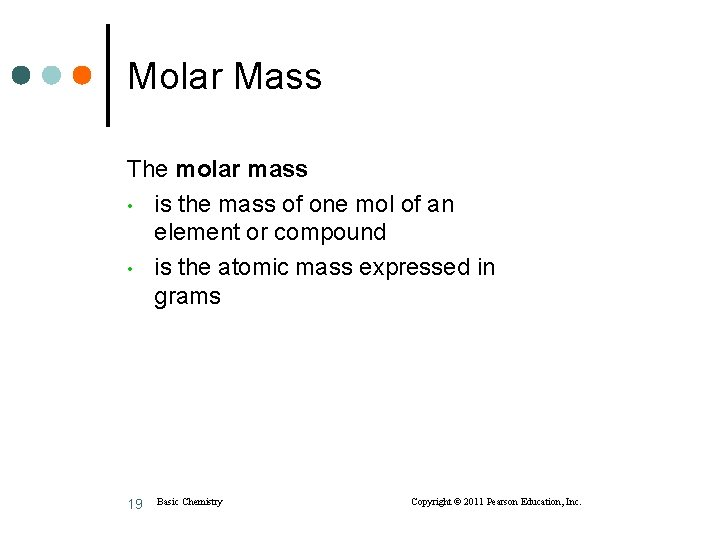Molar Mass The molar mass • is the mass of one mol of an element or compound • is the atomic mass expressed in grams 19 Basic Chemistry Copyright © 2011 Pearson Education, Inc.Molar Mass from the Periodic Table 20 Basic Chemistry Copyright © 2011 Pearson Education, Inc.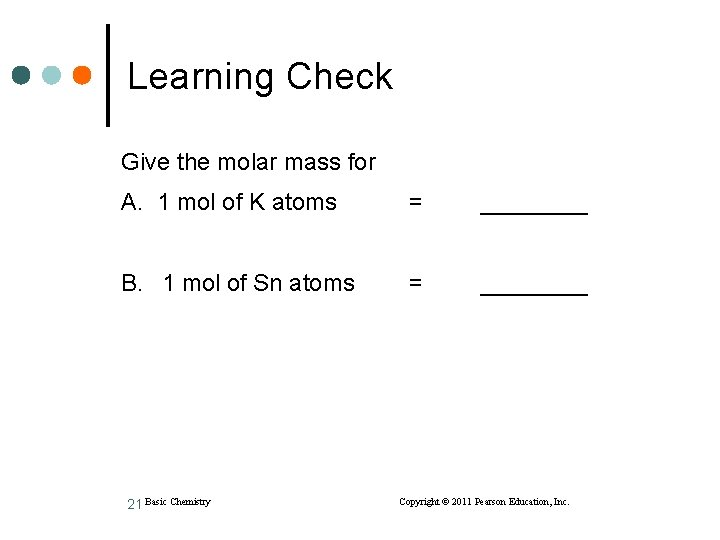Learning Check Give the molar mass for A. 1 mol of K atoms = ____ B. 1 mol of Sn atoms = ____ 21 Basic Chemistry Copyright © 2011 Pearson Education, Inc.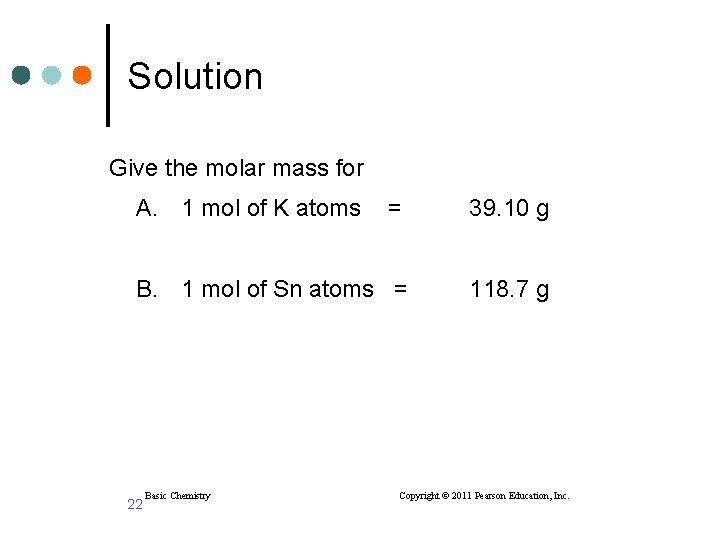Solution Give the molar mass for A. 1 mol of K atoms = 39. 10 g B. 1 mol of Sn atoms = 118. 7 g 22 Basic Chemistry Copyright © 2011 Pearson Education, Inc.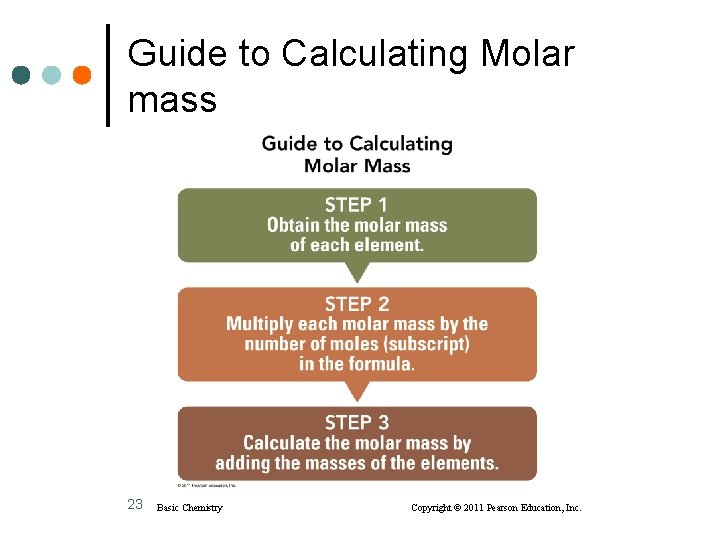Guide to Calculating Molar mass 23 Basic Chemistry Copyright © 2011 Pearson Education, Inc.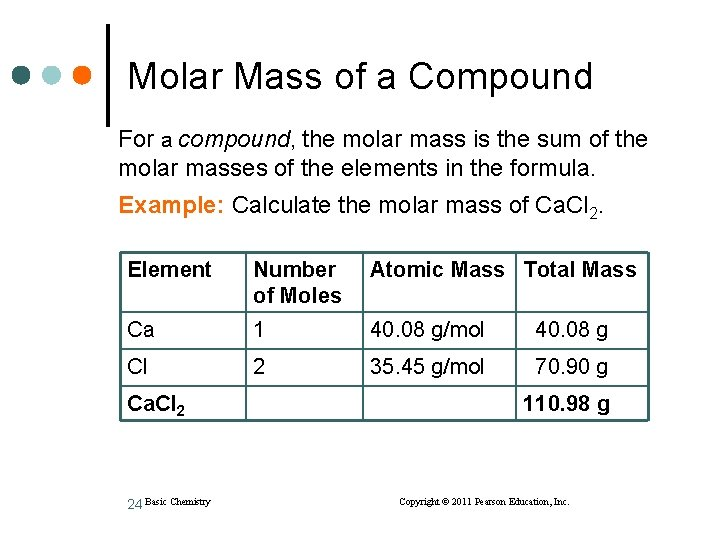Molar Mass of a Compound For a compound, the molar mass is the sum of the molar masses of the elements in the formula. Example: Calculate the molar mass of Ca. Cl 2. Element Number of Moles Atomic Mass Total Mass Ca 1 40. 08 g/mol 40. 08 g Cl 2 35. 45 g/mol 70. 90 g Ca. Cl 2 24 Basic Chemistry 110. 98 g Copyright © 2011 Pearson Education, Inc.Molar Mass of K 3 PO 4 Determine the molar mass of K 3 PO 4. Element Number of Atomic Mass Total Mass Moles in K 3 PO 4 K 3 39. 10 g/mol P 1 30. 97 g/mol 30. 97 g O 4 16. 00 g/mol 64. 00 g K 3 PO 4 25 Basic Chemistry 117. 3 g 212. 3 g Copyright © 2011 Pearson Education, Inc.Some One-Mol Quantities 26 Basic Chemistry Copyright © 2011 Pearson Education, Inc.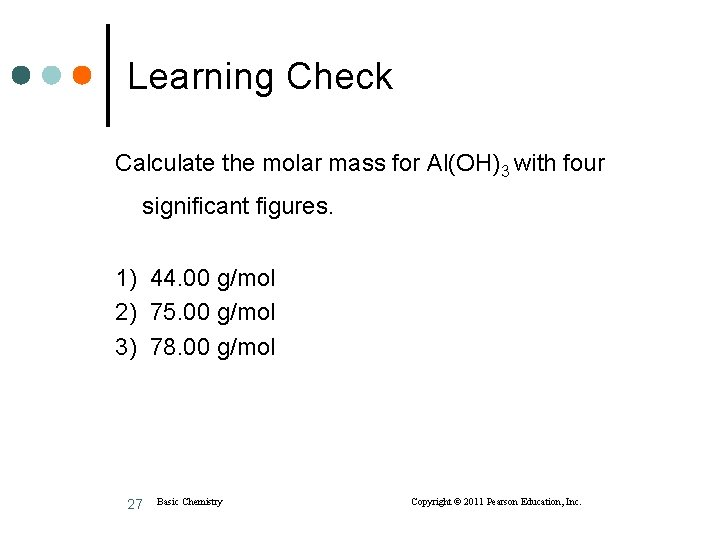Learning Check Calculate the molar mass for Al(OH)3 with four significant figures. 1) 44. 00 g/mol 2) 75. 00 g/mol 3) 78. 00 g/mol 27 Basic Chemistry Copyright © 2011 Pearson Education, Inc.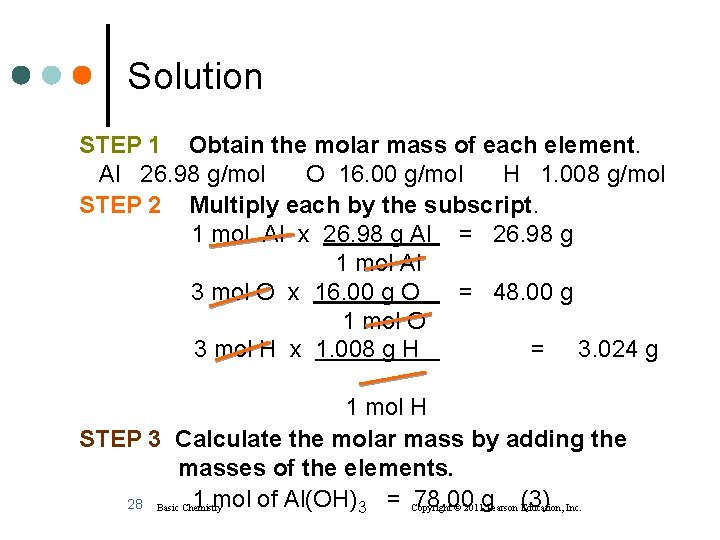Solution STEP 1 Obtain the molar mass of each element. Al 26. 98 g/mol O 16. 00 g/mol H 1. 008 g/mol STEP 2 Multiply each by the subscript. 1 mol Al x 26. 98 g Al = 26. 98 g 1 mol Al 3 mol O x 16. 00 g O = 48. 00 g 1 mol O 3 mol H x 1. 008 g H = 3. 024 g 1 mol H STEP 3 Calculate the molar mass by adding the masses of the elements. 1 mol of Al(OH)3 = Copyright 78. 00 28 Basic Chemistry © 2011 g Pearson (3) Education, Inc.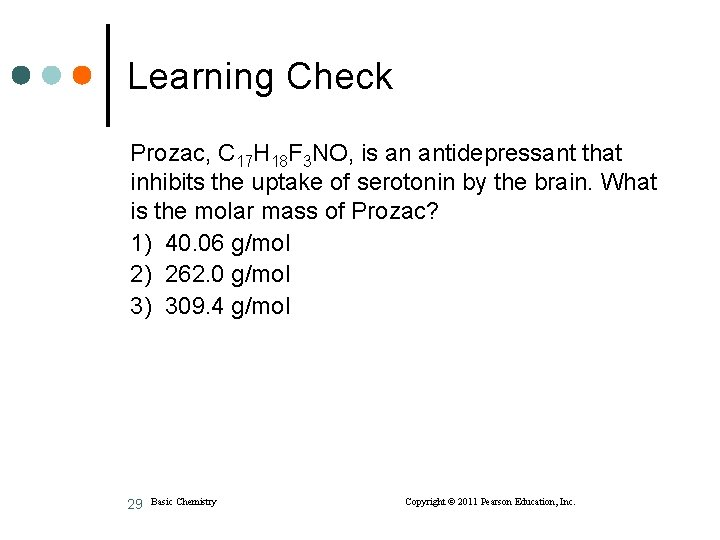Learning Check Prozac, C 17 H 18 F 3 NO, is an antidepressant that inhibits the uptake of serotonin by the brain. What is the molar mass of Prozac? 1) 40. 06 g/mol 2) 262. 0 g/mol 3) 309. 4 g/mol 29 Basic Chemistry Copyright © 2011 Pearson Education, Inc.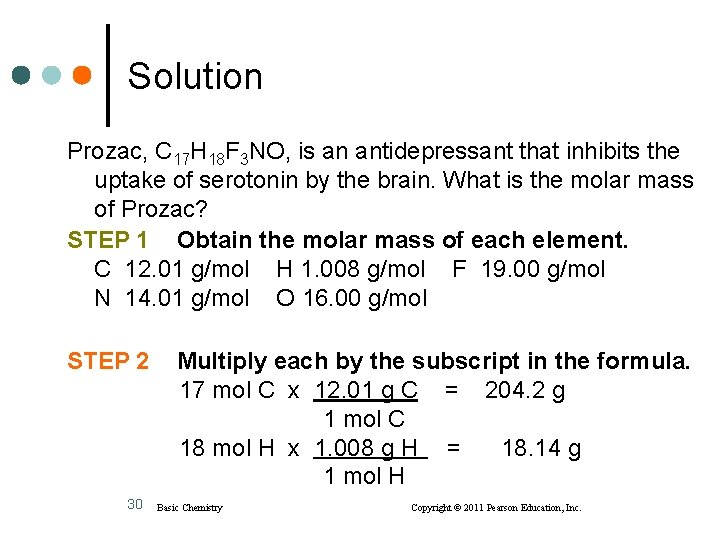Solution Prozac, C 17 H 18 F 3 NO, is an antidepressant that inhibits the uptake of serotonin by the brain. What is the molar mass of Prozac? STEP 1 Obtain the molar mass of each element. C 12. 01 g/mol H 1. 008 g/mol F 19. 00 g/mol N 14. 01 g/mol O 16. 00 g/mol STEP 2 30 Multiply each by the subscript in the formula. 17 mol C x 12. 01 g C = 204. 2 g 1 mol C 18 mol H x 1. 008 g H = 18. 14 g 1 mol H Basic Chemistry Copyright © 2011 Pearson Education, Inc.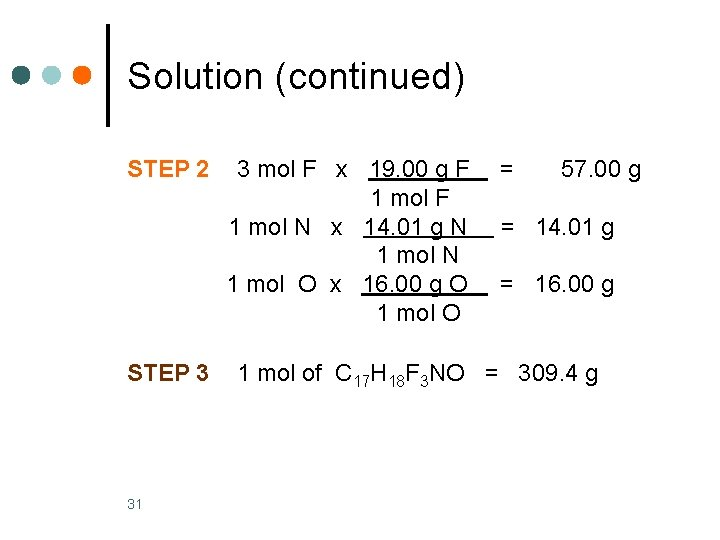Solution (continued) STEP 2 STEP 3 31 3 mol F x 19. 00 g F 1 mol N x 14. 01 g N 1 mol O x 16. 00 g O 1 mol O = 57. 00 g = 14. 01 g = 16. 00 g 1 mol of C 17 H 18 F 3 NO = 309. 4 g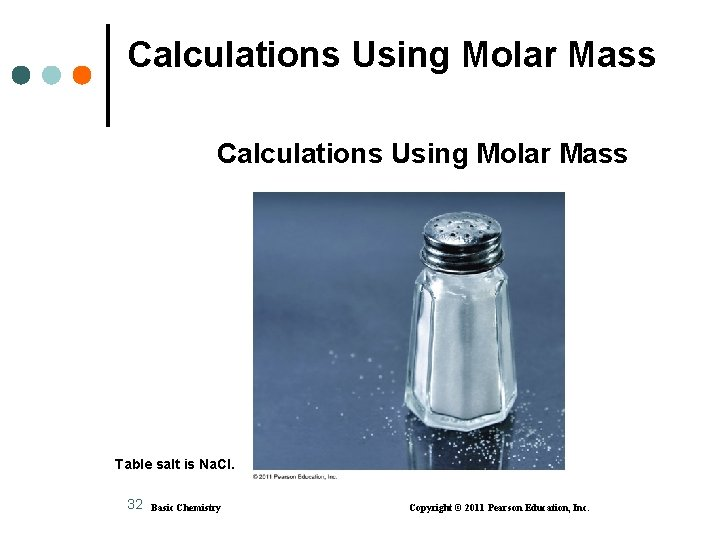Calculations Using Molar Mass Table salt is Na. Cl. 32 Basic Chemistry Copyright © 2011 Pearson Education, Inc.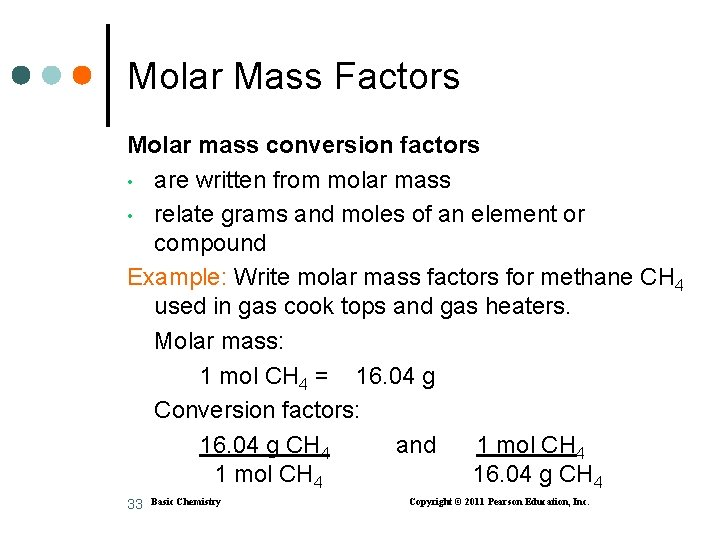Molar Mass Factors Molar mass conversion factors • are written from molar mass • relate grams and moles of an element or compound Example: Write molar mass factors for methane CH 4 used in gas cook tops and gas heaters. Molar mass: 1 mol CH 4 = 16. 04 g Conversion factors: 16. 04 g CH 4 and 1 mol CH 4 16. 04 g CH 4 33 Basic Chemistry Copyright © 2011 Pearson Education, Inc.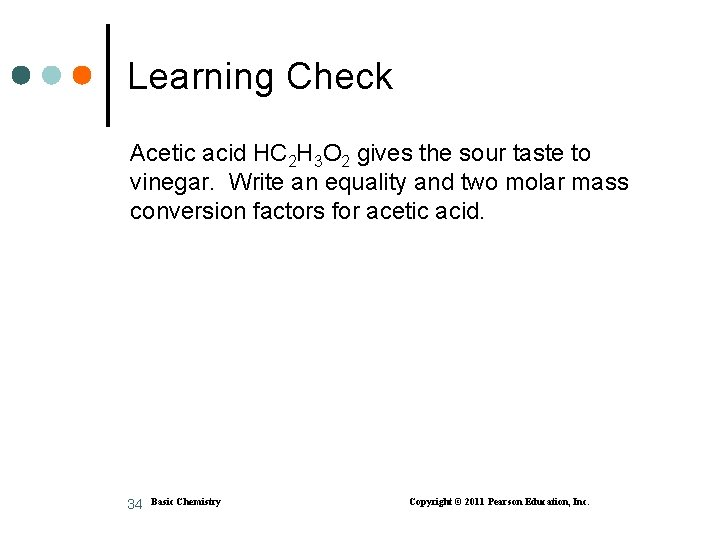Learning Check Acetic acid HC 2 H 3 O 2 gives the sour taste to vinegar. Write an equality and two molar mass conversion factors for acetic acid. 34 Basic Chemistry Copyright © 2011 Pearson Education, Inc.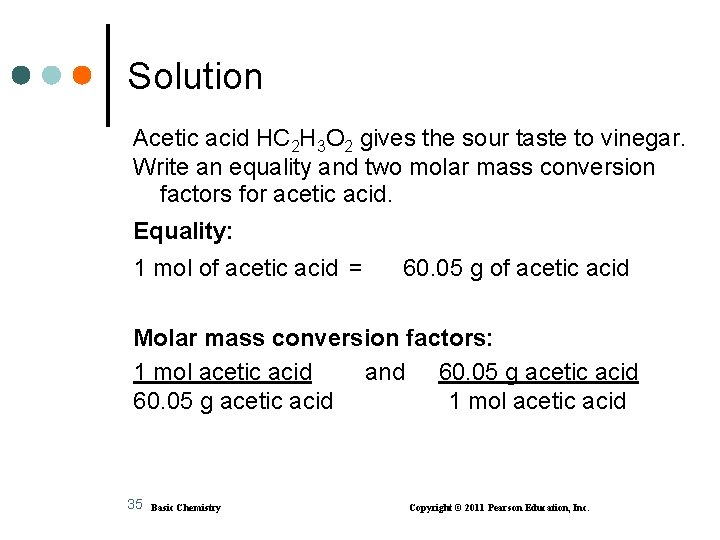Solution Acetic acid HC 2 H 3 O 2 gives the sour taste to vinegar. Write an equality and two molar mass conversion factors for acetic acid. Equality: 1 mol of acetic acid = 60. 05 g of acetic acid Molar mass conversion factors: 1 mol acetic acid and 60. 05 g acetic acid 1 mol acetic acid 35 Basic Chemistry Copyright © 2011 Pearson Education, Inc.Guide to Calculation Using Molar Mass 36 Basic Chemistry Copyright © 2011 Pearson Education, Inc.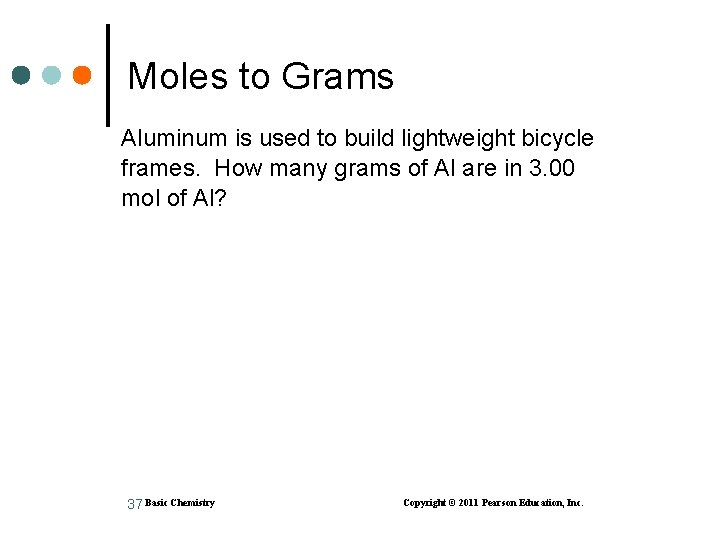Moles to Grams Aluminum is used to build lightweight bicycle frames. How many grams of Al are in 3. 00 mol of Al? 37 Basic Chemistry Copyright © 2011 Pearson Education, Inc.Solution STEP 1 Given 3. 00 mol of Al Need grams of Al STEP 2 Plan moles of Al grams of Al STEP 3 Equalities/Conversion Factors 1 mol of Al = 26. 98 g of Al 1 mol Al and 26. 98 g Al 1 mol Al 38 Basic Chemistry Copyright © 2011 Pearson Education, Inc.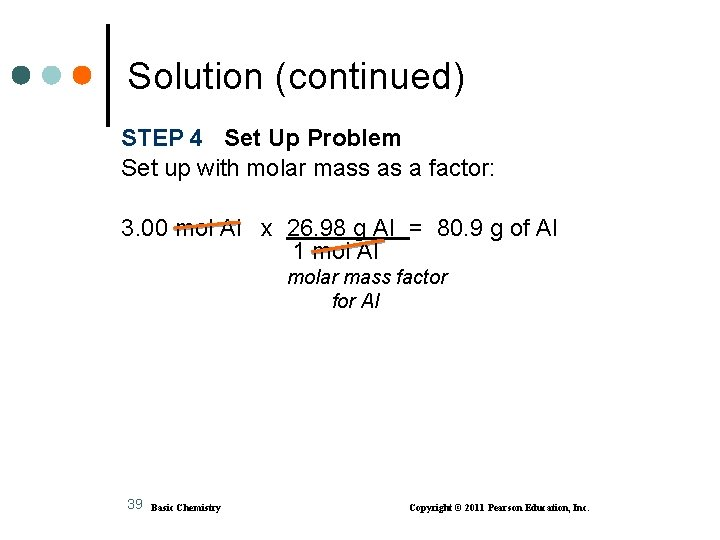Solution (continued) STEP 4 Set Up Problem Set up with molar mass as a factor: 3. 00 mol Al x 26. 98 g Al = 80. 9 g of Al 1 mol Al molar mass factor for Al 39 Basic Chemistry Copyright © 2011 Pearson Education, Inc.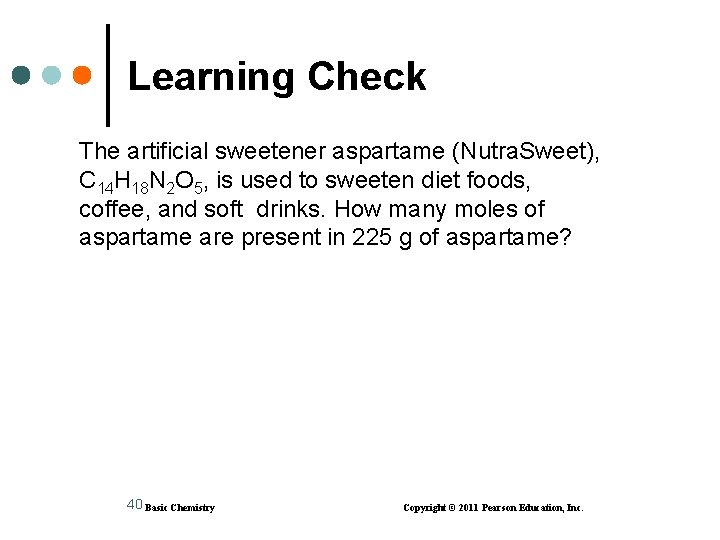Learning Check The artificial sweetener aspartame (Nutra. Sweet), C 14 H 18 N 2 O 5, is used to sweeten diet foods, coffee, and soft drinks. How many moles of aspartame are present in 225 g of aspartame? 40 Basic Chemistry Copyright © 2011 Pearson Education, Inc.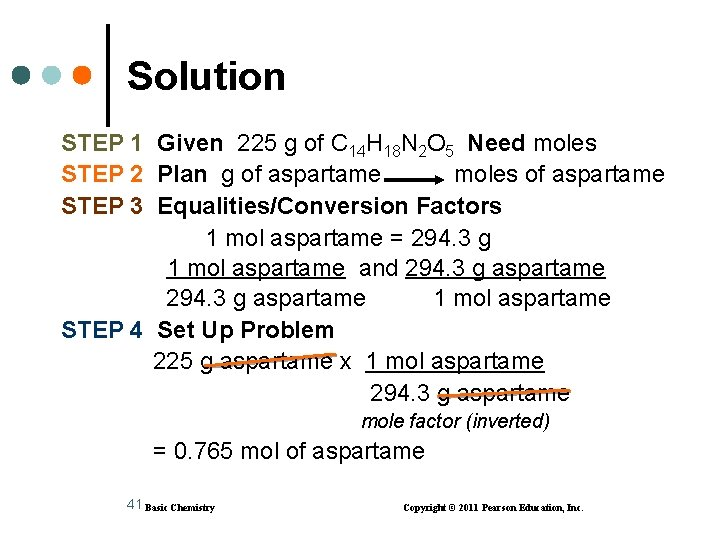Solution STEP 1 Given 225 g of C 14 H 18 N 2 O 5 Need moles STEP 2 Plan g of aspartame moles of aspartame STEP 3 Equalities/Conversion Factors 1 mol aspartame = 294. 3 g 1 mol aspartame and 294. 3 g aspartame 1 mol aspartame STEP 4 Set Up Problem 225 g aspartame x 1 mol aspartame 294. 3 g aspartame mole factor (inverted) = 0. 765 mol of aspartame 41 Basic Chemistry Copyright © 2011 Pearson Education, Inc.Learning Check Allyl sulfide, C 6 H 10 S, is a compound that has the odor of garlic. How many moles of C 6 H 10 S are in 225 g of C 6 H 10 S? 42 Basic Chemistry Copyright © 2011 Pearson Education, Inc.Solution STEP 1 Given 225 g of C 6 H 10 S Need moles of C 6 H 10 S STEP 2 Plan g of C 6 H 10 S moles of C 6 H 10 S STEP 3 Equalities/Conversion Factors 1 mol of C 6 H 10 S = 114. 21 g of C 6 H 10 S 1 mol C 6 H 10 S and 114. 21 g C 6 H 10 S 1 mol C 6 H 10 S STEP 3 Set Up Problem 225 g C 6 H 10 S x 1 mol C 6 H 10 S 114. 21 g C 6 H 10 S = 1. 97 mol C 6 H 10 S 43 Basic Chemistry Copyright © 2011 Pearson Education, Inc.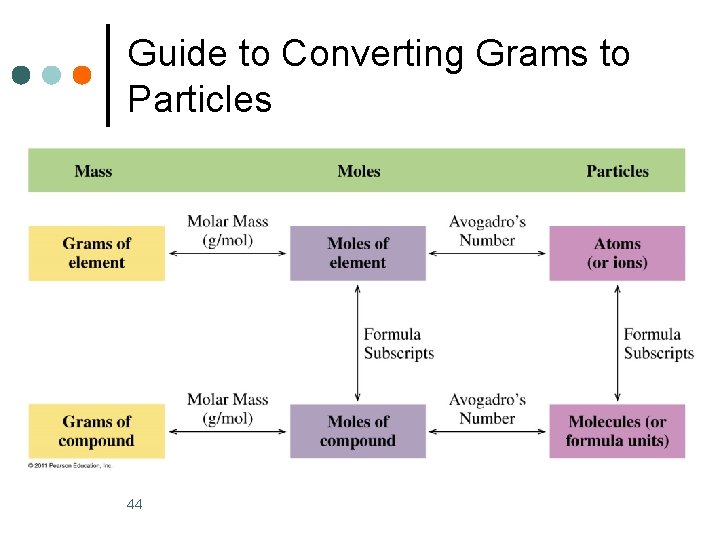Guide to Converting Grams to Particles 44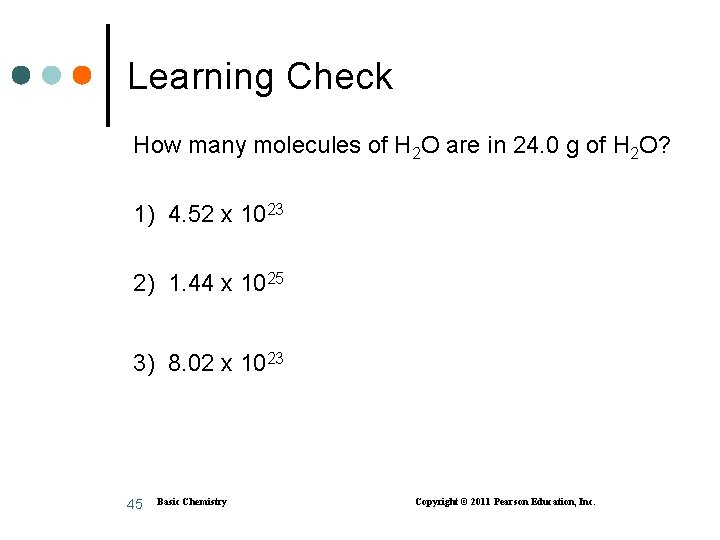Learning Check How many molecules of H 2 O are in 24. 0 g of H 2 O? 1) 4. 52 x 1023 2) 1. 44 x 1025 3) 8. 02 x 1023 45 Basic Chemistry Copyright © 2011 Pearson Education, Inc.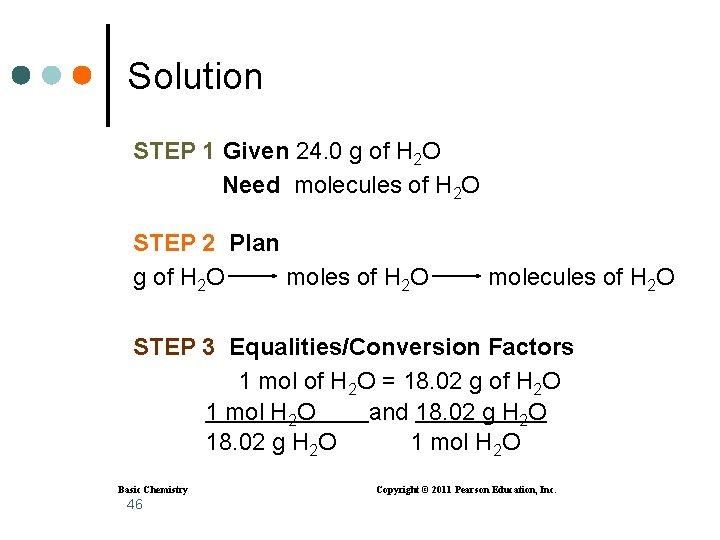Solution STEP 1 Given 24. 0 g of H 2 O Need molecules of H 2 O STEP 2 Plan g of H 2 O moles of H 2 O molecules of H 2 O STEP 3 Equalities/Conversion Factors 1 mol of H 2 O = 18. 02 g of H 2 O 1 mol H 2 O and 18. 02 g H 2 O 1 mol H 2 O Basic Chemistry 46 Copyright © 2011 Pearson Education, Inc.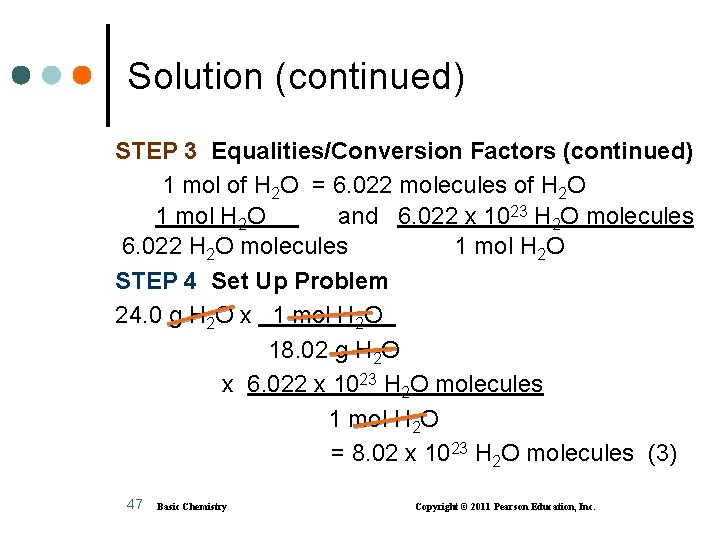Solution (continued) STEP 3 Equalities/Conversion Factors (continued) 1 mol of H 2 O = 6. 022 molecules of H 2 O 1 mol H 2 O and 6. 022 x 1023 H 2 O molecules 6. 022 H 2 O molecules 1 mol H 2 O STEP 4 Set Up Problem 24. 0 g H 2 O x 1 mol H 2 O 18. 02 g H 2 O x 6. 022 x 1023 H 2 O molecules 1 mol H 2 O = 8. 02 x 1023 H 2 O molecules (3) 47 Basic Chemistry Copyright © 2011 Pearson Education, Inc.